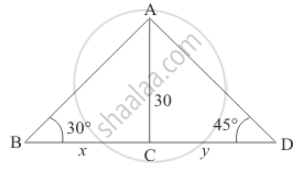Share

# From a Point on a Bridge Across a River, the Angles of Depression of the Banks on Opposite Side of the River Are 30° and 45° Respectively. If the Bridge is at the Height of 30 M from the Banks, Find the Width of the River. - CBSE Class 10 - Mathematics

#### Question

From a point on a bridge across a river, the angles of depression of the banks on opposite side of the river are 30° and 45° respectively. If the bridge is at the height of 30 m from the banks, find the width of the river.

#### Solution

Let BD be the width of the river. And the angle of depression of the bank on opposite side of the river is 30° and 45° respectively. It is given that AC = 30 m.

Let BC = x and CD = y. And ∠ABC = 30°, ∠ADC = 45°.

Here we have to find the width of the river.

We have the following figureSo we use trigonometric ratios.

In a triangle ABC

=> tan 30° = (AC)/(BC)

=> 1/sqrt3 = 30/x

=> x = 30sqrt3

=> tan 45° = (AC)/(CD)

=> 1 = 30/y

=> y = 30

So width of river is

x + y = 30sqrt3

x + y = 30(sqrt3 + 1)

Hence the width of river is (30(sqrt3 + 1)m

Is there an error in this question or solution?

#### Video TutorialsVIEW ALL 

Solution From a Point on a Bridge Across a River, the Angles of Depression of the Banks on Opposite Side of the River Are 30° and 45° Respectively. If the Bridge is at the Height of 30 M from the Banks, Find the Width of the River. Concept: Heights and Distances.
S